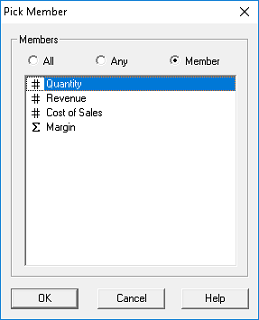Create a Constraint ExpressionSpecify the Member Name in a Constraint Expression:

1.   Open a slice (Use Sales Account by Month slice of the Avon Trading Company database).

2.   In the PowerOLAP ribbon, select Slice tab, chose Constrain Slice command.
The Edit Constraints dialog box is displayed, with the cursor defaulting in the Row Constraints text box.

3.   You may know what Members of the column Dimension you wish to constrain and can type the Member name without assistance. However, if you need prompting, and to ensure correct spelling, you can use the Pick button to pick the Members to which you want to apply a constraint.

The Member name specified must be a Member of the innermost (when nested) Dimension of the opposing axis you are constraining. For example, if you are in the Row Constraint box and you pick a Member, you will be picking a Member of the column Dimension to constrain. You can pick any Member or all Members of the opposing axis to constrain.4.   Select Quantity. Notice that the Member radio button is automatically selected.

5.   Double-click on Quantity or click OK to return to the Edit Constraint dialog box.

Your Pick Member choice returns the word ‘Quantity‘ into the Rows Constraint box.

The operator you select next will determine what you want to see in your Slice. If you write (or select, by double-clicking from the Operator list box) the ‘less than’ sign, <, followed by the value 5, you will get only rows where Quantity<5 in your slice. Alternately, writing the expression Quantity>5 will constrain your Slice to showing only rows where Quantity is greater than 5.

6.   For this example, finish the expression Quantity>5 and click OK.

7.   Press F9 to update the Slice.

The Slice view now shows rows where the Quantity values are all greater than 5.

Sample Constraints

The following expressions are further examples of constraints and their meanings:

• Quantity > 20
(“Keep the rows where the column values in Quantity is greater than 20″)
• Revenue= 2129
(“Keep the rows where the column values in Gross Profit is equal to 2129″)
• Cost of Sales <> 122.30
(“Keep the rows where the column values in Cost of Sales is not equal to 122.30″)
• Revenue top 4
(“Keep the rows where the column values are the top 4 values”)

If both the Regions dimension and Months dimension are in Rows and the Accounts dimension is in Columns and you want to constrain Columns, then the constraint Member needs to be a Member of the innermost Rows dimension Month.

For example:

• January < 2
(“Keep the columns where the row values in January is less than 2″)
• QTR1 > 20
(“Keep the columns where the row values in QTR1 is greater than 20″)
• March >= 100
(“Keep the columns where the row values in March is greater than or equal to 100″)
• Total Year bottom 15
(“Keep the columns where the row values in Total Year are the bottom 15 values”)

Any and All

Constraints using ‘Any’ or ‘All’ act on cells displayed along the opposing axis.

If a constraint is composed for Rows:

• Any < 100
(“Keep the rows where the column values in any cell is less than 100”)
• All <> 25
(“Keep the rows where the column values in all cells is not equal to 25”)

If a constraint is composed for Columns:

• Any < 100
(“Keep the columns where the row values in any cell is less than 100”)
• All <> 25
(“Keep the columns where the row values in all cells is not equal to 100”)

Combining Constraints Expressions via AND and OR

Constraint expressions can be combined using the Boolean operators AND and OR. Note that constraints joined by AND evaluate before other constraint expressions.

For example:

• Units < 2 AND ‘Gross Margin‘ > 20 OR Revenue <= 25
• Price <= 25 OR ‘Units’ > 20 OR Revenue <= 5

Constraint expressions may also be parenthetically grouped.

For example:

• Units < 2 AND (‘Gross Margin‘ > 20 OR Revenue <= 25)